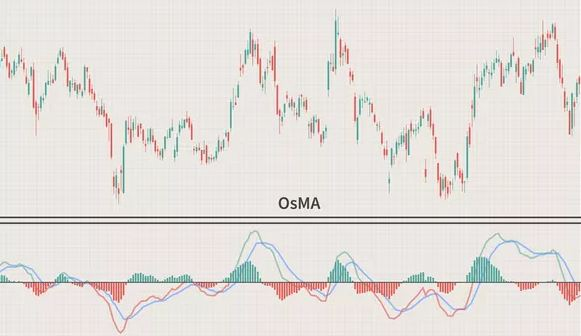## What is OsMA, and how to use it?

Jan 22 • Forex Trading Articles, Forex Trading Strategies • 662 Views • Comments Off on What is OsMA, and how to use it?

An oscillator is an indicator that exhibits a relationship between any two given set periods of the Moving Average. A trend indicator shows the fluctuations when an asset is either oversold or overbought.

The oscillator of moving average (OsMA) combines an oscillator and the moving average of that oscillator. It shows the difference between the two over a given period.

OsMA is commonly designed using the data of an indicator called MACD. Although any oscillator can be used for designing an OsMA indicator, it is commonly referred to as a modification of the MACD.

MACD uses a signal line for the display of the moving average. This signal line is the average of the MACD line. OsMA uses a histogram to display the difference between those lines of MACD to provide trend confirmation. The bigger the difference between signal lines and the histogram, the bigger will be the OsMA value.

## Adding Indicator to the Price Chart

For adding an indicator to the price chart, first, click on the Main Menu, then go to Insert -> Indicators -> Oscillators -> Moving Average of Oscillator.

## Formula for the OsMA

The formula for calculating OsMA value is:

OsMA = MACD – SMA

MACD = EMA12 – EMA26

Where MACD (Oscillator Value) is the value of MACD histogram, SMA (Simple Moving Average) is the signal line of MACD, and EMA is the Exponential Moving Averages.

## Parameters while installing OsMA indicator

The following parameters of OsMA should be set up while installing:

• Slow EMA is by default set to 26. This is the EMA with a larger period.
• Quick EMA is by default set to 12. This is the EMA with a shorter period.
• SMA is by default set to 9 and is the signal line of the MACD.

## Calculating the Oscillator of a Moving Average

• Select an oscillator. The time frame will be based on the oscillator used.
• Select the Moving Average type.
• Choose the number of periods in the chosen MA.
• Calculate the oscillator and MA value of the oscillator.
• Calculate the OsMA value using the formula (OsMA = MACD – SMA)
• Repeat steps 4 and 5 for each period.

## OsMA signals

The OsMA is a useful indicator for checking trends of the market and their strengths. It shows what is up in the market. The signals generated by the OsMA indicator are:

### Histogram above 0

If the histogram value is above 0, especially several numbers above 0, then this shows an uptrend and rising prices because of the overbought state of the market.

### Histogram below 0

If the histogram value is below 0, especially several numbers below 0, then this shows a downtrend and falling prices because of the oversold state of the market.

### Zero-line Crossover

A zero-line crossover occurs when the oscillator crosses above or below its moving average (MA). OsMA records a negative value indicating a falling price if the oscillator drops below its moving average.

On the other hand, OsMA records a positive value indicating a rising price if the oscillator goes above its moving average. Crossover is usually helpful in making good trades, but it can mislead a trader if the prices are choppy.

So, it is better to only consider the crossovers that align with the longer-term uptrend for prices.

## Bottom line

The OsMA indicator is a useful modification of MACD that is easy to use and shows trends of the market very well. It also gives signals earlier as compared to the classical MACD or others.